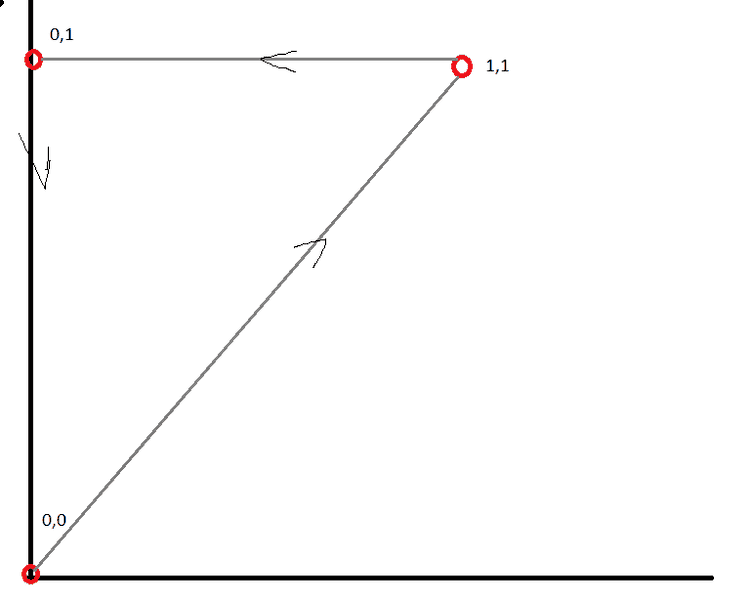# Limits of Integration of a Triangle

Dopplershift

## Homework Statement

Suppose you have a Triangle with the vertices, (0,0) (1,1) and (0,1). Integrating along that path.

I have some differential function dZ where Z = Z(x,y)

## The Attempt at a Solution

[/B]
If I need to integrate, then I need to find the limits of integration. Am I correct with the following.

0 < x < y (x is between x and y)
0 < y < 1 (y is between 0 and 1).

I have attached my awful MS Paint drawing to demonstrate the triangle.http://[url=https://ibb.co/dgJzMF][PLAIN]https://image.ibb.co/bP8Naa/trainglethemrmo.png [Broken][/url][/PLAIN]

Last edited by a moderator:

Homework Helper
Gold Member
This is a double integral, where the ends of the integrals need to change so that the integrals only cover the triangle. Start with a double integral with unknown ends and fill in the blanks:
??(∫??Z(x,y) dx)dy

I don't think that I should say more on a homework problem. Give it a try.

Dopplershift
How to I integrate if the integral is a sum such as dz = y dx + (x+2y)dy ?

And are my limits of integration correct?

Homework Helper
Gold Member
What are
How to I integrate if the integral is a sum such as dz = y dx + (x+2y)dy ?
I may have misunderstood. Are you integrating Z or dZ?
And are my limits of integration correct?
What are your limits? I don't see them.

Dopplershift
You didn't misunderstood, I mistyped, my apologies. It is dZ.

My limits I assumed are
0<y<1
0<x< y

Homework Helper
Gold Member
You need to put the integrand into the formula of the integrals with correct limits on the integrals and keep track of which integration has dx≡0 or dy≡0.

Dopplershift
You need to put the integrand into the formula of the integrals with correct limits on the integrals and keep track of which integration has dx≡0 or dy≡0.

That makes sense. Thank you! :)